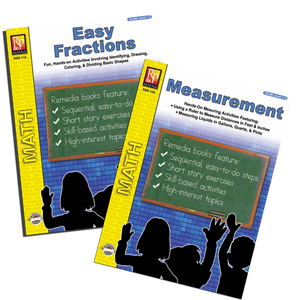Measurements and Fractions Practice – The Home of Times Tales®!The Home of Times Tales®!

# Measurements & Fractions Practice (2 Pack) - Grades 1-2

Regular price \$14.95
An Introduction to measurements and fractions!
From using the paper ruler provided to measure inches, to figuring pints, quarts and gallons, students will learn basic measuring through the 19 imaginative lessons in the book.

How long is the funny looking worm? How tall is the double-scoop ice cream cone? Given that 1 gallon = 4 quarts, color the number of quart containers you could fill with two gallons of milk.

Fully illustrated, the activities in this lesson unit will get your students excited about measuring all sorts of things from the “guitar” and “hammer” pictured in the book to the crayons on their desks. 24 pages.

An introduction to fractions!

Easy-to-grasp, graphically illustrated shapes are used to begin teaching the concept of fractions.

Example: A half-moon shape is divided into two even parts and students are asked to color the fraction «.

In all, there are 25 lessons in this teaching unit. As they work through the exercises, students will enjoy identifying, drawing, coloring, and dividing the basic shapes into fractions of 1/2, 1/4, 1/8, 1/3 and on and on.